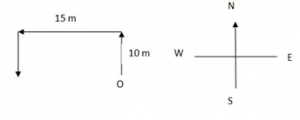## Practice Questions – Reasoning -1

1. 34 is related 12 in the same way as 59 is related to

(a) 45

(b) 14

(c) 42

(d) 38

(e) 47

2. What should come next in the following number sequence?

2 2 3 2 3 4 2 3 4 5 2 3 4 5 6 2 3 4 5 6

(a) 2

(b) 7

(c) 8

(d) Q

(e) None of these

3. Among P, Q, R, S, T and U, R is taller than only P and U. S is shorter than only T and Q. If each of them has a different height, who among them will be the third from top when they are arranged in descending order of their heights?

(a) R

(b) P

(c) S

(d) Q

(e) None of these

4. vikas walked 10 meter towards north, took a left turn and walked 15 meters, and again took a left turn and walked 10 meters and stooped walking. Towards which direction was he facing when he stopped walking?

(a) South

(b) South- west

(c) South-East

(d) Cannot be determined

(e) None of these

5. Four of the following five are alike in a certain way and so form a group. Which is the one that does not belong to that group?

(a) BD

(b) CE

(c) GI

(d) FH

(e) NL

6. How many such 7s are there in the following number sequence each of which is immediately preceded by 5 and not immediately followed by 8? 2 3 5 7 8 9 3 4 5 7 6 1 9 3 5 7 4 8 3 2 5 7 8 8 9 2 5

(a) None

(b) One

(c) Two

(d) three

(e) None of these

7. If it possible to form a number with the first, the fourth and the seventh digits of the number 4 6 7 1 3 5 8 which of the following will be the digit in the ten’s place of that two- digit odd number ? if no such number, can be formed, give O as the answer and if more than one such number can be made give X as the answer.

(a) 2

(b) 9

(c) 3

(d) O

(e) X

8. Among P, Q, R, S and T, Q is younger than only S and R and older than T. Who among them is the oldest?

(a) S

(b) R

(c) P

(e) None of these

9. In a certain code 59345 is written as \$AD%F and 8173 is written as HB#D. How is 9865 written in that code?

(a) HAF\$

(b) AFH\$

(d) BHF\$

(e) None of these

10. If ‘M’ denotes ‘÷’, ‘K’ denotes ‘-‘ ‘T’ denotes ‘×’ and ‘R’ denotes ‘+’ then __ 20 K 16 T 8 M 4 R 6 =?

(a) 18

(b) -6

(c) -12

(d) -18

(e) None of these

Explanation

1. The second number is the product of the digits of the first
2. 2/23/234/2345/23456/234567
3. Since S is shorter than only two persons (T and Q), he must be the third in descending order of height.
4.5. In all others the second letter comes two letters after the first in the alphabet.
6. 2 3  5  7  8   9  3  4  5  7  6  1  9  3  5  7  4  8  3  2  5  7  8  8   9  2  5
7. The digits are 4, 1 and 8 respectively. From this we get 841, which is the square of 29. Now, 29 has 2 in the ten’s place.
8. Either S or R.
9.

 5 9 3 4 6 8 1 7 \$ A D % F H B #

10.
20  K 16   T  8   M  4   R   6
→ 20 – 16 × 8 ÷ 4 + 6
→ 20 – 32 + 6 = -6